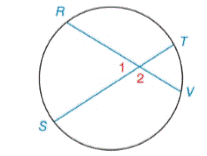Chapter 6.2, Problem 14E### Elementary Geometry for College St...

6th Edition
Daniel C. Alexander + 1 other
ISBN: 9781285195698

#### Solutions

Chapter
Section### Elementary Geometry for College St...

6th Edition
Daniel C. Alexander + 1 other
ISBN: 9781285195698
Textbook Problem
1 views

# Given: m ∠ 1 = 63 ° m R S ⌢ = 3 x + 6 m V T ⌢ = x Find: m R S ⌢Exercises 14, 15

To determine

To find:

mRS when m1=63°, mRS=3x+6,mVT=x

Explanation

Theorem:

The measure of an angle formed by two chords that intersect within a circle is one-half the sum of the measures of the arcs intercepted by the angle and its vertical angle.

Calculation:

Given,

mRS when m1=63°, mRS=3x+6,mVT=x

By theorem

m1=12(mRS+mVT)63°=12(3x+6+x)63°=12

### Still sussing out bartleby?

Check out a sample textbook solution.

See a sample solution

#### The Solution to Your Study Problems

Bartleby provides explanations to thousands of textbook problems written by our experts, many with advanced degrees!

Get Started

#### Evaluate the integral. 26. 0/4sec6tan6d

Single Variable Calculus: Early Transcendentals

#### limx0+(1+x)1/x= a) e b) e c) 1e d) 1e

Study Guide for Stewart's Single Variable Calculus: Early Transcendentals, 8th

#### True or False: is a convergent series.

Study Guide for Stewart's Multivariable Calculus, 8th

#### Define and compute the three measures of central tendency.

Research Methods for the Behavioral Sciences (MindTap Course List)